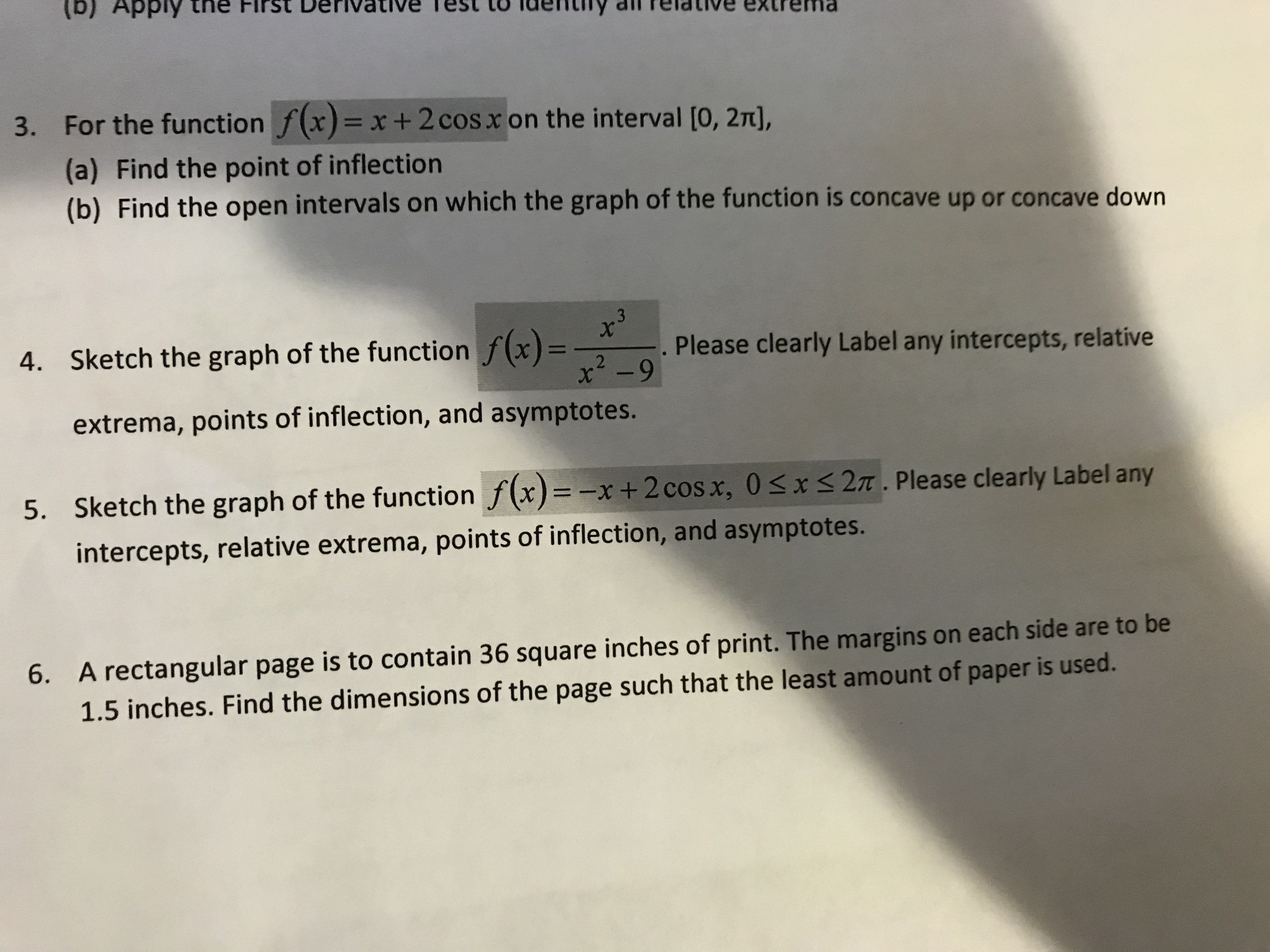# (b) Apply the FIrst Derivativeest to idelntny dl f emaPwanxa aAnPiau iuP3. For the function f(x)= x + 2 cos x on the interval [0, 27t],(a) Find the point of inflection(b) Find the open intervals on which the graph of the function is concave up or concave down4. Sketch the graph of the function f(x)=. Please clearly Label any intercepts, relativex2-9extrema, points of inflection, and asymptotes.5. Sketch the graph of the function f(x) -x + 2 cos x, 0

Question
30 viewshelp_outlineImage Transcriptionclose(b) Apply the FIrst Derivative est to id elntny dl f ema Pwanxa aAnPiau iuP 3. For the function f(x)= x + 2 cos x on the interval [0, 27t], (a) Find the point of inflection (b) Find the open intervals on which the graph of the function is concave up or concave down 4. Sketch the graph of the function f(x)= . Please clearly Label any intercepts, relative x2-9 extrema, points of inflection, and asymptotes. 5. Sketch the graph of the function f(x) -x + 2 cos x, 0
check_circle

Step 1

Consider the given function,

Step 2

Axis interception points of the given function

y-intercept is the point on the graph where x=0

Step 3

Asymptotes of the given fun...help_outlineImage TranscriptioncloseThe function -x+2cos x has no undefined points. So there is no asymptotes for the function fullscreen

### Want to see the full answer?

See Solution

#### Want to see this answer and more?

Solutions are written by subject experts who are available 24/7. Questions are typically answered within 1 hour.*

See Solution
*Response times may vary by subject and question.
Tagged in

### Calculus